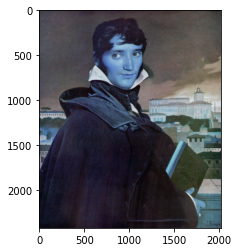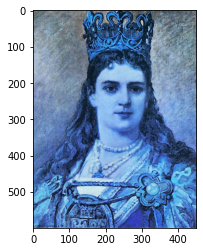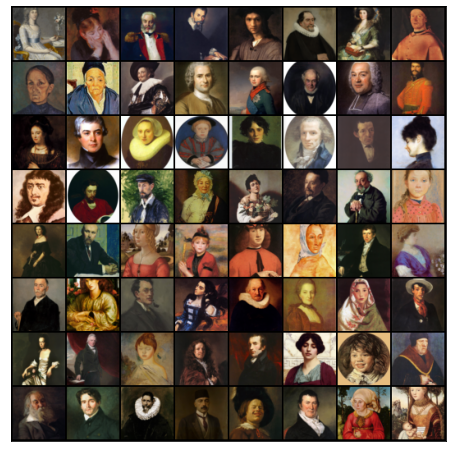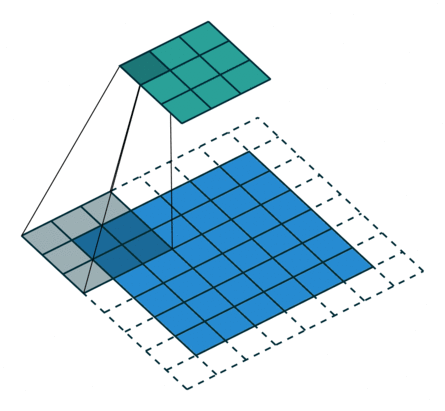Learn data science and machine learning by building real-world projects on Jovian

What is Generative adversarial networks (GANs)?

Generative adversarial networks (GANs) are an exciting recent innovation in machine learning. GANs are generative models: they create new data instances that resemble your training data. For example, GANs can create images that look like photographs of human faces, even though the faces don't belong to any real person.

These images were created by a GAN:This notebook is a minimum demo for GAN. Since PaperSpace allows maximum run time limit of 6 hrs in free tier, we will only train a lightweight model in this notebook.

Background: What is a Generative Model?

What does "generative" mean in the name "Generative Adversarial Network"? "Generative" describes a class of statistical models that contrasts with discriminative models. Informally:

• Generative models can generate new data instances.
• Discriminative models discriminate between different kinds of data instances.
in other words There are two neural networks: a Generator and a Discriminator.
• The generator generates a latent vector(random vector/random tensor/random matrix)
• the discriminator attempts to detect whether a given sample is "real" (picked from the training data) or "fake" (generated by the generator).Training happens in tandem: we train the discriminator for a few epochs, then train the generator for a few epochs, and repeat. This way both the generator and the discriminator get better at doing their jobs.

Folder Structure

. /root ├── Training GAN on gpu.ipynb ├── art-portraits │ └── Portraits └── image-1 └── image-2 └── image-n

objective

modeling objective Generative modeling is an unsupervised learning task in machine learning that involves automatically discovering and learning the regularities or patterns in input data in such a way that the model can be used to generate or output new examples that plausibly could have been drawn from the original dataset.
Let's install required libraries
# Uncomment and run the appropriate command for your operating system, if required
# No installation is reqiured on Google Colab / Kaggle notebooks

# Linux / Binder / Windows (No GPU)

# Linux / Windows (GPU)

# MacOS (NO GPU)
# !pip install numpy matplotlib torch torchvision torchaudio
!pip install opendatasets

opendatasets
import opendatasets as od
dataset_url = 'https://www.kaggle.com/karnikakapoor/art-portraits'
Let's Set our data directory
import os

DATA_DIR = 'art-portraits'
print(os.listdir(DATA_DIR))
['Portraits']
Exploring the Data
print(os.listdir(DATA_DIR+'/Portraits')[:10])
['bc1cf30d88ee3291af791f77eac70582c.jpg', 'fe2f7028ade1f27ceddbb15d41a28717c.jpg', 'c74063dc222e6a9a0cbd59ab5bfec9cdc.jpg', 'ecbc995cc283ecf84a2eae0348a786e6c.jpg', 'bb06e5b6cc1e8bb99aa0e92766053c56c.jpg', '5f207999aa8fa9c36ef5e806acf1ffedc.jpg', 'b09e6ab959a1f9500ce8d2e1912c24f9c.jpg', '1b90bcd2a313c074920760ba5968c742c.jpg', '43a37fc480f85ddde62a41962e45e082c.jpg', '7bd7db51e351630d26c5943623f127bdc.jpg']
import cv2
import numpy as np
import matplotlib.pyplot as plt
def show_jpg(jpg_name):
plt.imshow(img)
plt.show()
show_jpg(DATA_DIR+'/Portraits/'+'bc1cf30d88ee3291af791f77eac70582c.jpg')show_jpg('art-portraits/Portraits/00ca56f16c0bae52185ea31f95f0484cc.jpg')The dataset has a single folder called images which contains all 4,000+ images in JPG format.
Let's load this dataset using the ImageFolder class from torchvision. We will also resize and crop the images to 64x64 px, and normalize the pixel values with a mean & standard deviation of 0.5 for each channel. This will ensure that pixel values are in the range (-1, 1), which is more convenient for training the discriminator. We will also create a data loader to load the data in batches.
from torchvision.datasets import ImageFolder
import torchvision.transforms as T
image_size = 64
batch_size = 128
stats = (0.5, 0.5, 0.5), (0.5, 0.5, 0.5)
train_ds = ImageFolder(DATA_DIR, transform=T.Compose([
T.Resize(image_size),
T.CenterCrop(image_size),
T.ToTensor(),
T.Normalize(*stats)]))

train_dl = DataLoader(train_ds, batch_size, shuffle=True, num_workers=3, pin_memory=True)
Let's create helper functions to denormalize the image tensors and display some sample images from a training batch.
import torch
from torchvision.utils import make_grid
import matplotlib.pyplot as plt
%matplotlib inline
def denorm(img_tensors):
return img_tensors * stats + stats
def show_images(images, nmax=64):
fig, ax = plt.subplots(figsize=(8, 8))
ax.set_xticks([]); ax.set_yticks([])
ax.imshow(make_grid(denorm(images.detach()[:nmax]), nrow=8).permute(1, 2, 0))

def show_batch(dl, nmax=64):
for images, _ in dl:
show_images(images, nmax)
break
show_batch(train_dl)Using a GPU To seamlessly use a GPU, if one is available, we define a couple of helper functions (get_default_device & to_device) and a helper class DeviceDataLoader to move our model & data to the GPU, if one is available.
def get_default_device():
"""Pick GPU if available, else CPU"""
if torch.cuda.is_available():
else:

def to_device(data, device):
"""Move tensor(s) to chosen device"""
if isinstance(data, (list,tuple)):
return [to_device(x, device) for x in data]
return data.to(device, non_blocking=True)

"""Wrap a dataloader to move data to a device"""
def __init__(self, dl, device):
self.dl = dl
self.device = device

def __iter__(self):
"""Yield a batch of data after moving it to device"""
for b in self.dl:
yield to_device(b, self.device)

def __len__(self):
"""Number of batches"""
return len(self.dl)
Based on where you're running this notebook, your default device could be a CPU (torch.device('cpu')) or a GPU (torch.device('cuda')).
device = get_default_device()
device
device(type='cuda')
We can now move our training data loader using DeviceDataLoader for automatically transferring batches of data to the GPU (if available).

Discriminator Network

The discriminator is a classifier that determines if the input samples are real or fake. These input samples can be real samples coming from the training data, or fake coming from the generator. We'll use a convolutional neural networks (CNN) which outputs a single number output for every image We'll use stride of 2 to progressively reduce the size of the output feature map, and we're using the Leaky ReLU activation for the discriminator.import torch.nn as nn
discriminator = nn.Sequential(
# in: 3 x 64 x 64

nn.Conv2d(3, 64, kernel_size=4, stride=2, padding=1, bias=False),
nn.BatchNorm2d(64),
nn.LeakyReLU(0.2, inplace=True),
# out: 64 x 32 x 32

nn.Conv2d(64, 128, kernel_size=4, stride=2, padding=1, bias=False),
nn.BatchNorm2d(128),
nn.LeakyReLU(0.2, inplace=True),
# out: 128 x 16 x 16

nn.Conv2d(128, 256, kernel_size=4, stride=2, padding=1, bias=False),
nn.BatchNorm2d(256),
nn.LeakyReLU(0.2, inplace=True),
# out: 256 x 8 x 8

nn.Conv2d(256, 512, kernel_size=4, stride=2, padding=1, bias=False),
nn.BatchNorm2d(512),
nn.LeakyReLU(0.2, inplace=True),
# out: 512 x 4 x 4

nn.Conv2d(512, 1, kernel_size=4, stride=1, padding=0, bias=False),
# out: 1 x 1 x 1

nn.Flatten(),
nn.Sigmoid())
Let's move the discriminator model to the chosen device.
discriminator = to_device(discriminator, device)

Generator Network

The input to the generator is typically a vector or a matrix of random numbers (referred to as a latent tensor) which is used as a seed for generating an image. The generator will convert a latent tensor of shape (128, 1, 1) into an image tensor of shape 3 x 28 x 28. To achive this, we'll use the ConvTranspose2d layer from PyTorch, which is performs to as a transposed convolution (also referred to as a deconvolution). Learn morelatent_size = 128
generator = nn.Sequential(
# in: latent_size x 1 x 1

nn.ConvTranspose2d(latent_size, 512, kernel_size=4, stride=1, padding=0, bias=False),
nn.BatchNorm2d(512),
nn.ReLU(True),
# out: 512 x 4 x 4

nn.ConvTranspose2d(512, 256, kernel_size=4, stride=2, padding=1, bias=False),
nn.BatchNorm2d(256),
nn.ReLU(True),
# out: 256 x 8 x 8

nn.ConvTranspose2d(256, 128, kernel_size=4, stride=2, padding=1, bias=False),
nn.BatchNorm2d(128),
nn.ReLU(True),
# out: 128 x 16 x 16

nn.ConvTranspose2d(128, 64, kernel_size=4, stride=2, padding=1, bias=False),
nn.BatchNorm2d(64),
nn.ReLU(True),
# out: 64 x 32 x 32

nn.ConvTranspose2d(64, 3, kernel_size=4, stride=2, padding=1, bias=False),
nn.Tanh()
# out: 3 x 64 x 64
)
xb = torch.randn(batch_size, latent_size, 1, 1) # random latent tensors
fake_images = generator(xb)
print(fake_images.shape)
show_images(fake_images)
torch.Size([128, 3, 64, 64])